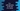# How to add elements to the end of a vector in C++ using push_back## How to add elements to the end of a vector in C++ using push_back:

Vector is like a dynamic array. The size of a vector changes automatically once we add an element to it.

Vector class provides a couple of useful methods. push_back method is used to add a new element to a vector at the end. It automatically increases the size of the vector by 1. In this post, we will learn how to use push_back with its definition and different examples.

### Definition of push_back:

push_back is defined as like below:

``v.push_back(e)``

Here, v is the vector to which we are adding the element e.

#### Return value of push_back:

It returns nothing or none.

### Example of push_back:

Let’s learn how push_back works with an example:

``````#include <iostream>
#include <vector>

using namespace std;

void printVector(vector<int> intVector)
{
for (vector<int>::iterator i = intVector.begin(); i != intVector.end(); i++)
{
cout << *i << " ";
}
}

int main()
{
vector<int> intVector{1, 2, 3, 4, 5};

intVector.push_back(6);
intVector.push_back(7);
intVector.push_back(8);

printVector(intVector);

return 0;
}``````

Here,

• intVector is a vector of integers.
• printVector method is used to print the content of an integer vector.
• We are using push_back to add 6, 7 and 8 to the end of the vector and printing its content.

If you run this program, it will print:

``1 2 3 4 5 6 7 8 ``

## push_back to insert multiple elements at the end of a vector:

push_back can be used to add only one element at a time. If you want to use it to add multiple elements, you need to use a loop. The loop will iterate through the elements and add each to the end of a vector.

``````#include <iostream>
#include <vector>

using namespace std;

void printVector(vector<int> intVector)
{
for (vector<int>::iterator i = intVector.begin(); i != intVector.end(); i++)
{
cout << *i << " ";
}
}

int main()
{
vector<int> intVector{1, 2, 3, 4, 5};
int givenNumbers = {6, 7, 8, 9};

for (int i = 0; i < 4; i++)
{
intVector.push_back(givenNumbers[i]);
}

printVector(intVector);

return 0;
}``````

In this program, we are using push_back to add all the numbers of the array givenNumbers to the end of the vector intVector.

We are using a for loop for that. Without a loop, we can’t add all of these numbers at one go.

If you run this program, it will print the below output:

``1 2 3 4 5 6 7 8 9``

i.e. the numbers of the array are added to the back of the vector.

### push_back to add vector into a vector:

We can create a vector that can hold other vectors. These types of vectors are also called vectors of vectors. We can use push_back to insert a vector to the end of a vector of vectors.

For example:

``````#include <iostream>
#include <vector>

using namespace std;

void printVector(vector<int> intVector)
{
for (vector<int>::iterator i = intVector.begin(); i != intVector.end(); i++)
{
cout << *i << " ";
}
}

int main()
{
vector<vector<int>> v{{1}, {2, 3}, {4, 5, 6}};

v.push_back({7, 8, 9, 10});

for (vector<vector<int>>::iterator i = v.begin(); i != v.end(); i++)
{
printVector(*i);
cout<<endl;
}

return 0;
}``````

For this example,

• v is a vector of vectors. It holds vectors that can hold integers.
• It uses push_back to add another vector to the end of v.
• The for loop is iterating through the vectors of v and internally it calls the printVector method to print the content of the vector elements.

If you run this program, it will print the below output:

``````1
2 3
4 5 6
7 8 9 10``````